# Forces in Action

## Friction

Friction is a force that occurs between two surfaces which are in contact and trying to move past each other. Friction acts in the opposite direction to the motion. Energy used against friction is transformed to heat. The temperature of the object and surface will increase.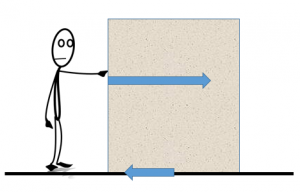## Work Done

Work done is a measurement of how much energy is required to move an object. It can be calculated using the equation (you DO need to remember this equation for your exam):

W = Fs

W = work done (J)

F = force (N)

__s __= distance (m)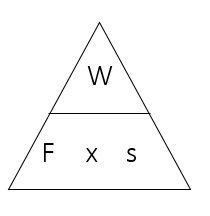Worked Example

A race up Ben Nevis starts in a car park 50m above sea level. The top of Ben Nevis is 1344m. Chris weighs 600N and Alison weighs 400N. Calculate the work done by each of them during the race.

1. Distance climbed = 1294m
2. Chris: work done = 600 x 1294 = 776,400 J
3. Alison: work done = 400 x 1294 = 517,600J
4. Chris needs more energy for the climb because he weighs more

## Elasticity

1. A force can cause an object to change shape
2. Elastic materials store Elastic Potential Energy
3. The EPE is released when the object springs back to it’s normal shape

For example, in a catapult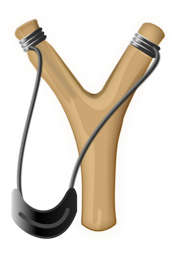1. Work done/energy used to stretch catapult
2. EPE stored in the catapult while it is stretched
3. EPE released (transformed into Kinetic Energy) when the catapult is released

Or, in a squash ball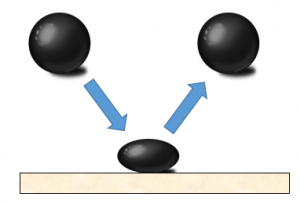1. Work done/energy used to squash ball
2. EPE stored in the ball while it is squashed
3. EPE released (transformed into Kinetic Energy) when the ball is released

## Hooke’s Law

The extension of an elastic object is proportional to the force acting on it.

This can be represented by the equation (you DO need to remember this equation for your exam):

1. __F __= k x e
2. __F __= force (N)
3. k = spring constant (N/m)
4. e = extension (m)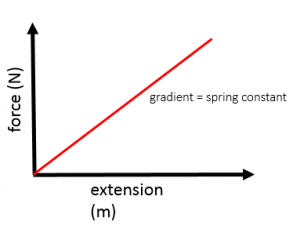1. The extension of an elastic object is proportional to the force acting on it until it reaches it’s elastic limit (limit of proportionality)
2. After the elastic limit, the object no longer returns to it’s original shape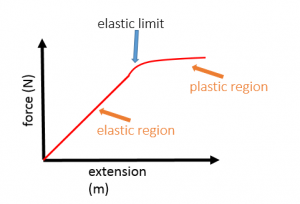## Required Practical – Elasticity

You need to be familiar with the practical method to investigate the relationship between force and extension (Hooke’s law)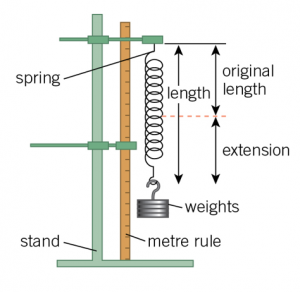Using an elastic material, such as a spring, add masses to the spring and measure the extension with each mass added.

Plotting a graph of force and extension should produce a directly proportional line as shown in the Hooke’s law section above. The gradient of the line is equal to the spring constant of the spring used In the experiment.

Possible causes of error in the experiment include:

1. Inaccurate measurement of the length or extension due to human error
2. Stretching the spring past it’s elastic limit, at which point the relationship between force and extension is no longer proportional and the spring does not return to it’s original size when the masses are removed

## Vector Diagrams

The resultant force on an object is is the sum of all the forces acting on an object. If the forces acting on the object are not all acting on the same line, vector diagrams can be used to find the resultant force.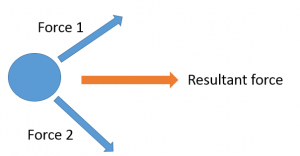Vector diagrams are __drawn to scale. __The length of the arrow equals the size of the force (eg 2cm = 2N).Draw first force to scale.Draw second force at the end of the other (using the same scale and at the correct angle)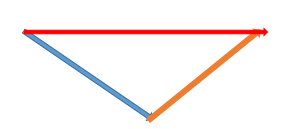The resultant force is shown by the line from the starting point to the ending point of your diagram

Worked Example

A tow rope is attached to a car at two points 1m apart at 35o to each other. If the pull on each attachment is 3000N, what is the force on the main tow rope?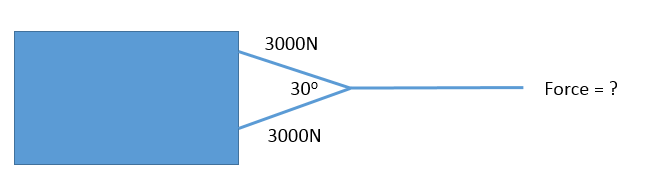Choose appropriate scale (eg 1cm = 1000N)Draw first force to scale (3cm = 3000N)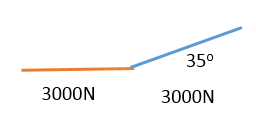Draw second force at the end of the previous force (at 35o )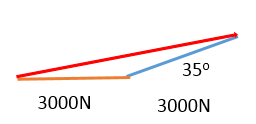Measure resultant line (5.7cm)

Resultant force = 5700N

## Stopping Distances

Vehicles do not stop instantly. During the time they take to stop, they are still travelling. This distance travelled is known as the stopping distance.

The stopping distance is made up of the thinking distance and the braking distance:

1. Thinking Distance - The distance travelled in the time it takes the driver to react
2. Braking Distance - The distance travelled after the brakes have been pressed
3. Stopping Distance = Thinking distance + braking distance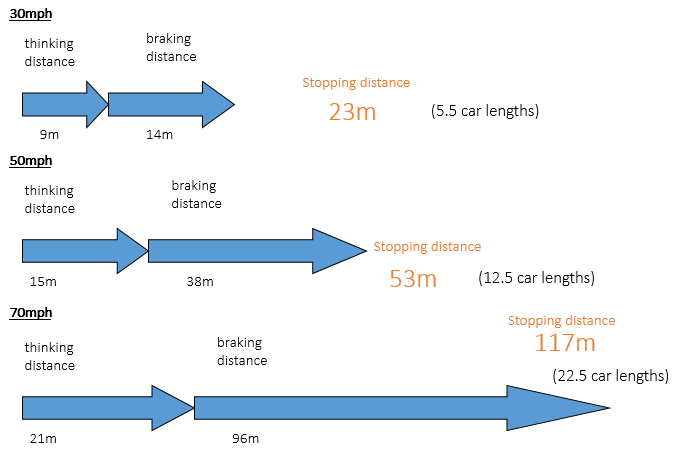Factors affecting thinking distance:

1. Alcohol/Drugs
2. Tiredness
3. Age
4. Speed
5. Distractions may prevent the driver stopping as quickly but have not affected his/her reaction time directly

Factors affecting braking distance:

1. Brake condition
2. Surface
3. Tyres
4. Weather – ice/rain
5. Speed

Thinking distance increases in linear relationship with speed. Thinking distance = speed x time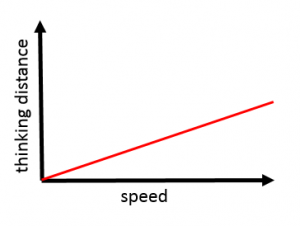Braking distance increases in a squared relationship with speed. If the speed doubles, the braking distance increases 4 times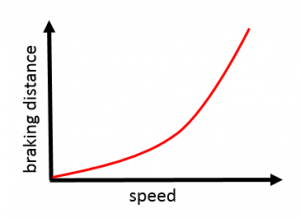## Resolving Forces

A force acting at an angle can be resolved into two perpendicular forces. Draw a scale diagram, with sides perpendicular to each other and with the original force as the diagonal. If drawn to scale, the sides of the rectangle can be measured to find the size of the force.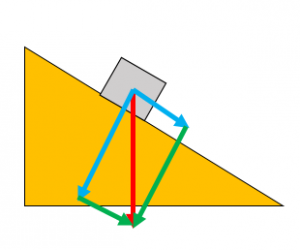Worked Example

A car of weight 10kN is parked on an uphill road. The road is inclined at an angle of 20o. Find the component of the car’s weight that acts down the slope.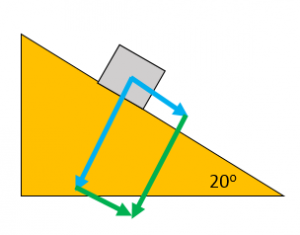Complete rectangle with original force as the diagonal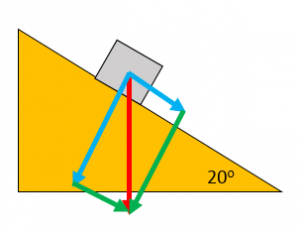Measure the component down the slope

Component down the slope = 3kN

A man pushes a car with a force of 550N for 12m. How much work was done?